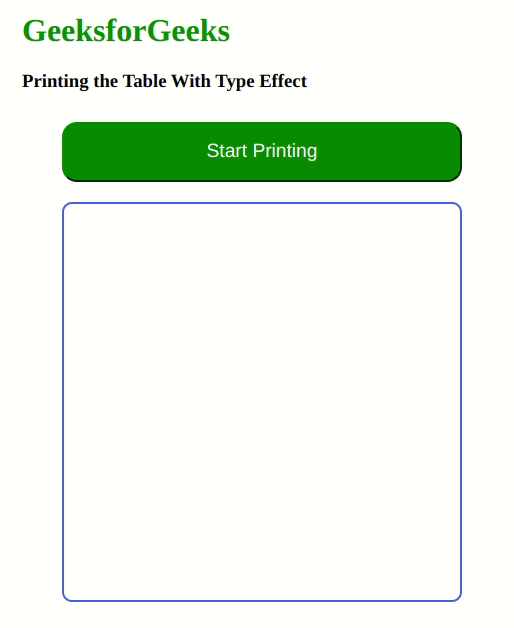Open in App
Not now

# Printing the table on the web page having type effect repeatedly

• Difficulty Level : Medium
• Last Updated : 24 Jan, 2023

In this article, we will see how to print the table on the web page having type effect repeatedly using HTML, CSS & Javascript. Here, the printing of the table with the type effect can be done with the JavaScript setTimeout() function that helps to set the timer to run the function or piece of code once the timer expires.

Syntax: The format for printing the table will be as given below:

```n * 1 = n
n * 2 = 2n
...```

Approach: The below approach will be implemented to print the table with type effect:

• Create a button with id as start & class as common, in order to include the different styling properties.
• Create a div section with id as a message.
• For styling the button, set the margin, width & height of the button, along with adding the background color as green. In order to make rounded corners, add the border-radius property to the button.
• For styling the <div> element, set the display property to flex & the setting the flex-direction as a row. Set the width & height of the div element, along with setting the value for justify-content as the center, in order to make the box shape to print the table inside of that box.
• For printing the table repeatedly, we have used the event Listener concept, along with using the different selectors in HTML DOM. Add the “click” event listener to the button, which will handle the printing of the table when the button is clicked.
• Create a function with the name typeEffect that will print every data of the table from the array in a row in the given interval of time using the setTimeOut() function.
• Call the function by clicking the button that was created in the above step.

Example: This example illustrates printing the table on the web page having a type effect repeatedly using HTML, CSS & JS.

## HTML

 ` ` `<``html` `lang``=``"en"``> ` ` `  `<``head``> ` `    ``<``meta` `charset``=``"UTF-8"` `/> ` `    ``<``meta` `name``=``"viewport"` `          ``content="``width``=``device``-width,  ` `                         ``initial-scale``=``1``.0" /> ` `    ``<``title``> ` `        ``Printing the table on the web page ` `        ``having type effect repeatedly ` `    `` ` ` `  `    ``<``style``> ` `        ``* { ` `            ``box-sizing: border-box; ` `        ``} ` ` `  `        ``h1 { ` `            ``color: green; ` `        ``} ` ` `  `        ``#message { ` `            ``display: flex; ` `            ``flex-direction: row; ` `            ``width: 400px; ` `            ``height: 400px; ` `            ``border: 2px solid royalblue; ` `            ``border-radius: 10px; ` `            ``color: royalblue; ` `            ``font-size: x-large; ` `            ``justify-content: center; ` `            ``padding: 30px; ` `            ``margin-left: 40px; ` `        ``} ` ` `  `        ``.common { ` `            ``width: 400px; ` `            ``height: 60px; ` `            ``margin: 10px 10px 20px 40px; ` `            ``background-color: rgba(0, 120, 0, 1); ` `            ``border-radius: 15px; ` `            ``border-color: rgba(0, 120, 0, 1); ` `            ``font-size: larger; ` `            ``color: white; ` `        ``} ` ` `  `        ``#start:hover { ` `            ``background-color: rgba(0, 160, 0, 1); ` `        ``} ` ` `  `        ``#stop:hover { ` `            ``background-color: rgba(0, 160, 0, 1); ` `        ``} ` `    `` ` ` ` ` `  `<``body``> ` `    ``<``h1``>GeeksforGeeks ` `    ``<``h3``> ` `        ``Printing the Table With Type Effect ` `    `` ` `    ``<``button` `id``=``"start"`  `            ``class``=``"common"``> ` `            ``Start Printing ` `    `` ` `    ``<``div` `id``=``"message"``> ` `    ``<``script``> ` `        ``const messageContainer =  ` `            ``document.getElementById("message"); ` `        ``const startButtton =  ` `            ``document.getElementById("start"); ` `        ``const typeSpeed = 150; ` `        ``let count = 1; ` `        ``let index = 0; ` `        ``function typeEffect(value) { ` ` `  `            ``// Set of values that will be displayed in the row ` `            ``let arr =  ` `                ``[value, " * ", count, " = ", value * count, "<``br``>"]; ` `            ``if (count >= 1 && count <= 10) { ` `                 `  `                ``// Appending the table inside the div element ` `                ``// having id messageContainer ` `                ``messageContainer.innerHTML += `\${arr[index]} `; ` `                ``index++; ` `                ``index = index % arr.length; ` `                ``if (index == 0) { ` `                    ``count++; ` `                ``} ` `                ``setTimeout(function () { ` `                    ``typeEffect(value); ` `                ``}, typeSpeed); ` `            ``} ` `            ``if (count == 11) { ` `                ``count = 1; ` `                ``messageContainer.textContent = ""; ` `            ``} ` `        ``} ` `        ``startButtton.addEventListener("click", function (e) { ` `            ``typeEffect(value = 10); ` `        ``}) ` `    `` ` ` ` ` `  ``

Output:My Personal Notes arrow_drop_up
Related Articles